# Taylor Series

A Taylor Series is an expansion of some function into an infinite sum of terms, where each term has a larger exponent like x, x2, x3, etc.

### Example: The Taylor Series for ex

ex = 1 + x + x22! + x33! + x44! + x55! + ...

says that the function:ex
is equal to the infinite sum of terms:1 + x + x2/2! + x3/3! + ... etc

(Note: ! is the Factorial Function.)

Does it really work? Let's try it:

### Example: ex for x=2

Well, we already know the answer is e2 = 2.71828... × 2.71828... = 7.389056...

But let's try more and more terms of our infinte series:

Terms   Result
1+2   3
1+2+222!   5
1+2+222!+233!   6.3333...
1+2+222!+233!+244!   7
1+2+222!+233!+244!+255!   7.2666...
1+2+222!+233!+244!+255!+266!   7.3555...
1+2+222!+233!+244!+255!+266!+277!   7.3809...
1+2+222!+233!+244!+255!+266!+277!+288!   7.3873...

It starts out really badly, but it then gets better and better!

Try using "2^n/fact(n)" and n=0 to 20 in the Sigma Calculator and see what you get.

Here are some common Taylor Series:

 Taylor Series expansion As Sigma Notation ex = 1 + x + x22! + x33! + ...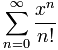sin x = x − x33! + x55! − ...cos x = 1 − x22! + x44! − ...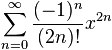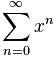(There are many more.)

## Approximations

We can use the first few terms of a Taylor Series to get an approximate value for a function.

Here we show better and better approximations for cos(x). The red line is cos(x), the blue is the approximation (try plotting it yourself) :

 1 − x2/2!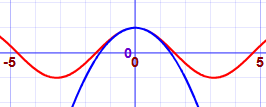1 − x2/2! + x4/4!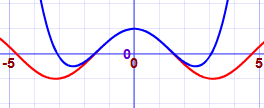1 − x2/2! + x4/4! − x6/6!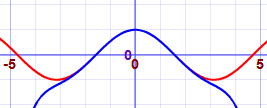1 − x2/2! + x4/4! − x6/6! + x8/8!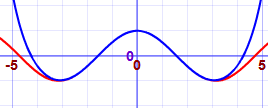You can also see the Taylor Series in action at Euler's Formula for Complex Numbers.

## What is this Magic?

How can we turn a function into a series of power terms like this?

Well, it isn't really magic. First we say we want to have this expansion:

f(x) = c0 + c1(x-a) + c2(x-a)2 + c3(x-a)3 + ...

Then we choose a value "a", and work out the values c0 , c1 , c2 , ... etc

And it is done using derivatives (so we must know the derivative of our function)### Quick review: a derivative gives us the slope of a function at any point.

These basic derivative rules can help us:

• The derivative of a constant is 0
• The derivative of ax is a (example: the derivative of 2x is 2)
• The derivative of xn is nxn-1 (example: the derivative of x3 is 3x2)

We will use the little mark to mean "derivative of".

OK, let's start:

To get c0, choose x=a so all the (x-a) terms become zero, leaving us with:

f(a) = c0

So c0 = f(a)

To get c1, take the derivative of f(x):

f(x) = c1 + 2c2(x-a) + 3c3(x-a)2 + ...

With x=a all the (x-a) terms become zero:

f(a) = c1

So c1 = f(a)

To get c2, do the derivative again:

f(x) = 2c2 + 3×2×c3(x-a) + ...

With x=a all the (x-a) terms become zero:

f(a) = 2c2

So c2 = f(a)/2

In fact, a pattern is emerging. Each term is

• the next higher derivative ...
• ... divided by all the exponents so far multiplied together (for which we can use factorial notation, for example 3! = 3×2×1)

And we get:

f(x) = f(a) + f'(a)1!(x-a) + f''(a) 2! (x-a)2 + f'''(a) 3! (x-a)3 + ...

Now we have a way of finding our own Taylor Series:

For each term: take the next derivative, divide by n!, multiply by (x-a)n

### Example: Taylor Series for cos(x)

f(x) = f(a) + f'(a) 1! (x-a) + f''(a) 2! (x-a)2 + f'''(a) 3! (x-a)3 + ...

The derivative of cos is −sin, and the derivative of sin is cos, so:

• f(x) = cos(x)
• f'(x) = −sin(x)
• f''(x) = −cos(x)
• f'''(x) = sin(x)
• etc...

And we get:

cos(x) = cos(a) sin(a) 1! (x-a) cos(a) 2! (x-a)2 + sin(a) 3! (x-a)3 + ...

Now put a=0, which is nice because cos(0)=1 and sin(0)=0:

cos(x) = 1 0 1! (x-0) 1 2! (x-0)2 + 0 3! (x-0)3 + 1 4! (x-0)4 + ...

Simplify:

cos(x) = 1 − x2/2! + x4/4! − ...